Latest Banking jobs   »

# Quantitative Aptitude Quiz For Bank Foundation 2023 – 24thMarch

Directions (1-5): In the following questions there are two equations given. You have to solve both the equations and give answer:
(a) if x > y
(b) if x < y
(c) if x ≥ y
(d) if x ≤ y
(e) if x = y or relation between x and y cannot be established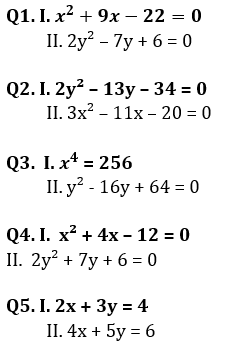Directions (6- 10): In each of these questions, two equations (I) and (II) are given. You have to solve both the equations and give answer
(a) if x>y
(b) if x≥y
(c) if x<y
(d) if x ≤y
(e) if x = y or no relation can be established between x and y.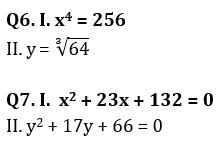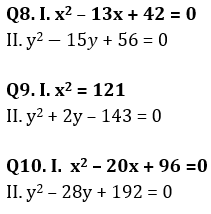Directions (11-15): In each of the following questions two equations are given. Solve these equations and give answer:
(a) if x≥y
(b) if x>y
(c) if x≤y
(d) if x<y
(e) x=y or no relation can be established between x and y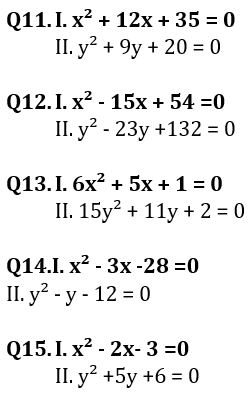Solutions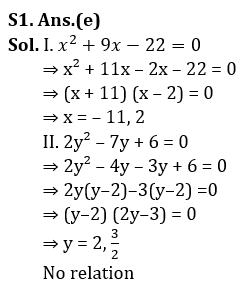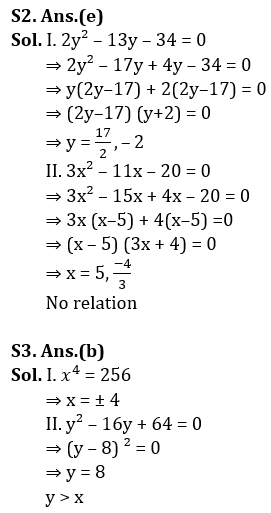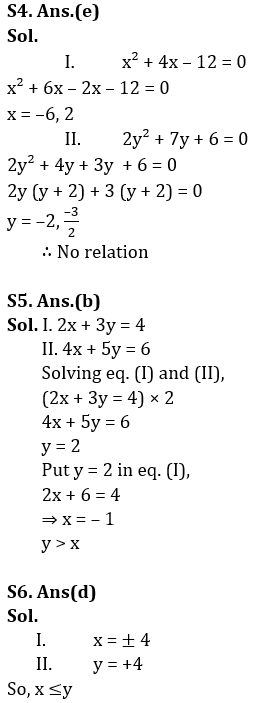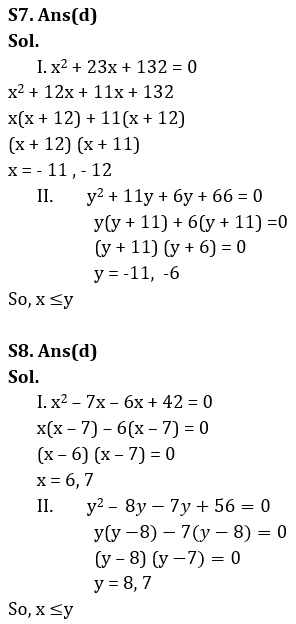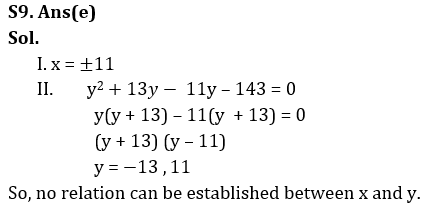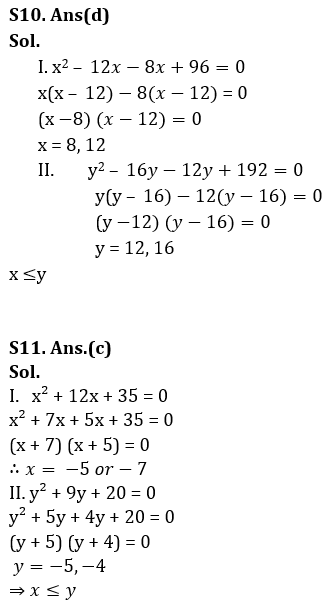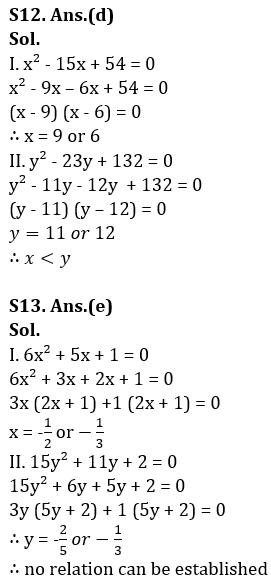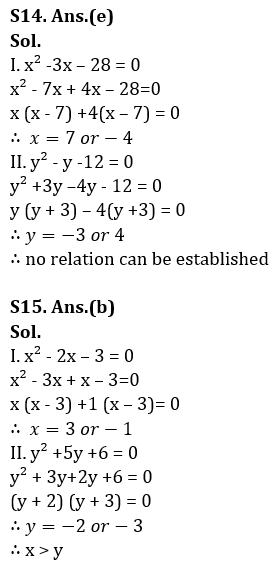.

## FAQs

### What is the selection process of the Bank Clerk?

The selection process of the Bank Clerk is Prelims & Mains.

#### Congratulations!Union Budget 2023-24: Free PDF